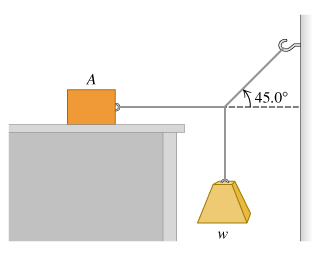# Problem: Block A in the figure weighs 65.1 N . The coefficient of static friction between the block and the surface on which it rests is 0.28. The weight w is 11.3 N and the system is in equilibrium.(a) Find the friction force exerted on block A.(b) Find the maximum weight for which the system will remain in equilibrium.

###### FREE Expert Solution
79% (493 ratings)
###### Problem Details

Block A in the figure weighs 65.1 N . The coefficient of static friction between the block and the surface on which it rests is 0.28. The weight w is 11.3 N and the system is in equilibrium.
(a) Find the friction force exerted on block A.
(b) Find the maximum weight for which the system will remain in equilibrium.Frequently Asked Questions

What scientific concept do you need to know in order to solve this problem?

Our tutors have indicated that to solve this problem you will need to apply the Equilibrium in 2D concept. You can view video lessons to learn Equilibrium in 2D. Or if you need more Equilibrium in 2D practice, you can also practice Equilibrium in 2D practice problems.

How long does this problem take to solve?

Our expert Physics tutor, Julia took 6 minutes and 51 seconds to solve this problem. You can follow their steps in the video explanation above.